APP Information

 Download Version 3.0.1 (14) Apk Size 3.61 MB App Developer VD Malware Check TRUSTED Install on Android 4.1.x and up App Package com.vd.mathsformulafree.apk MD5 d834ed5694f09bc9a198c4be8e660c6e Rate 5 Website http://gamesnapps4u.com/maths-formula-reference/App Description

A complete guide to maths formulas and concepts which is helpful in competitive like MBA , CAT , GMAT etc  and curriculum exams .
The Complete Reference of Maths Formulas covering the following topics
* Trigonometry Formulas
Right Angled Definition
Pythagoras Theorem
Tangent Identitites
Even Odd Formulas
Multi angle formulas
Double Angle Formulas
Inverse Trigonometric Functions
Trigonometric Ratios
*Geometry Formulas
Basics and Definitions
Triangles
Areas and Circumference
Volumes and Surface Area
*Number System Formulas
Remainder
Unit Digit
Last 2 Digits
*Divisibility Rules
*Algebra Formulas
*Exponents Formulas
*Logarithms Formulas
*Progressions Formulas
*Squares , cubes and roots Formulas
This maths formulas collection is useful for MBA exams , competitive and even curriculum exam .
It lists out all the important maths formulas/topics in Algebra, Geometry, Trigonometry. Regular review of these maths formulas and concepts will definitely help improve your grades.
Write us at [email protected] for suggestions and feedback for improvements.

App ChangeLog

• App Optimizations

More Android Apps to Consider

1.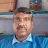Pankaj Kumar Ravi

Not given geometry all formulas. Example square and Cube

2.KUFRE ETIM

Very useful for quick reference

3.Sylvia Bendavid

Everything in one place! Thank you!

4.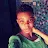Nara Agyei

This is the very experience for student

5.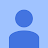vasu Kumar

good app for study.nothing else...

6.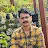Roshan Budathoki

It is nice app

7.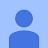It is great app

8.All are here

9.Justice Quainoo

Interested

10.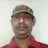Anil Kumar

Best